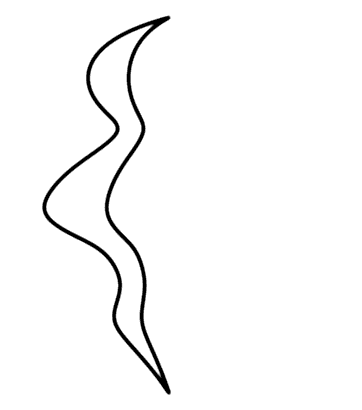OutputTextLayerAOutputTextLayerB
 The 3D symmetrical curves can be rotated by dragging the mouse. Two different 3D symmetrical interpretations produced with the same 2D curve that was used in Figure 4.4. Left: The slant and tilt of the symmetry plane of the 3D interpretation were set to 40° and 0°, respectively. Each of the two symmetrical halves of the 3D curve is planar (flat). Right: The slant and tilt of the symmetry plane were set to 40° and -25°, respectively. Neither of these two symmetrical halves of the 3D curve is planar (flat). The original image does not look like a 2D projection of this 3D symmetrical interpretation. See text for explanation.